IIT JAM FollowGarima Kaushik @Eduncle posted an Article
March 25, 2019 • 3:59 pm

# Matrix and Determinant - Eduncle Study Notes with Formulas, Questions & Solutions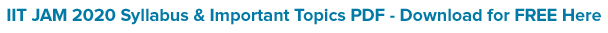Matrices and Determinants is a very important topic in Mathematics. Although it is taught to students from 12th to Graduation Level, in this article we'll provide you step by step lessons, MCQs and Numerical of IIT JAM Level (M.Sc.). Get Eduncle's study notes with formulas, questions and solutions to know what are Matrix and Determinant and how to solve these questions.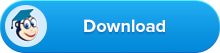In this brain-friendly guide, you'll study and quickly grasp following concepts:

1. Introduction - What are Matrix and Determinants?

2. Matrix and Determinant (All Formulas)

3. Theory

4. Practice Questions

4.1 Basic Level Questions (3 Questions)

4.2 Challenging Questions (3 Questions)

4.3 Question asked in IIT JAM from Matrix and Determinant (5 Questions)

5. Related topics to be studied before reading Matrix and Determinant.

So, load your brain with these important concepts! After completing all the topics, try solving the following questions and you will easily score full marks from this unit. Answers to these questions are given in the end. You can verify your answers and check your level of understanding.

## What Are Matrix and Determinants? - Introduction

The knowledge of matrices and determinants applies to several branches of science, as well as different mathematical discipline. Matrices are one of the most powerful tools in mathematics. It simplifies our work to a great extent when compared with other direct methods. The transformation of concept of matrices results in obtaining compact and simple methods of solving system of linear equations.

### Applications:

We use Matrices not only for the representation of the coefficients in system of linear equations, but it’s uses far exceeds.

The notation and operations of Matrices are used in electronic spreadsheet programs for personal computer, which can be further used in different areas of business and science like budgeting, sales projection, cost estimation, analyzing the results of an experiment etc.

Also, many physical operations such as magnification, rotation and reflection through a plane can be represented mathematically by matrices.

Matrices are also used in cryptography.

This mathematical tool is not only used in certain branches of sciences, but also in genetics, economics, sociology, modern psychology and industrial management.

For IIT JAM this topic is comparatively easy and scoring. If you want to get this theory lesson in PDF format, then hit the download button below:## Matrix and Determinants (All Formulas)

Any matrix A and its transpose both have the same Eigen values.

The trace of the matrix equals to the sum of the Eigen values of a matrix.

The determinant of the matrix A equals to the product of the Eigen values of A.

If λ1, λ2, ....., λn are the n-Eigen values of A, then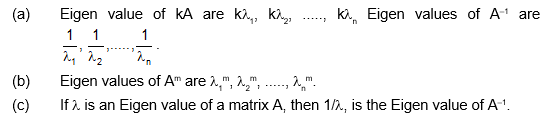Cayley Hamilton Theorem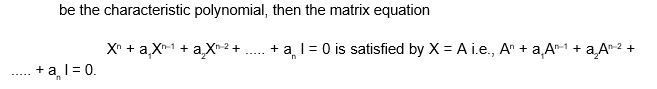A be an nxn matrix and let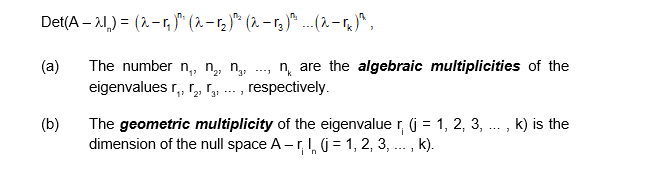Matrix exponential for a square matrix A,Download all the formulas for Matrix and determinants, eigen values, cayley hamilton theorem, matrix exponential etc. by clicking on the download button below.### Description

A matrix (plural matrices) is an ordered rectangular table of elements (or entries). The numbers or functions are called the elements or the entries of the matrix.

We denote matrices by capital letters.

For example: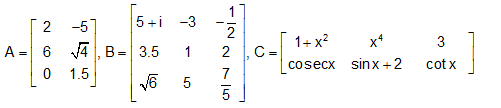In the above examples, the horizontal lines of elements are called rows of the matrix and the vertical lines of elements are called columns of the matrix. Thus A has 3 rows and 2 columns, B has 3 rows and 3 columns while C has 2 rows and 3 columns.

### Order of a matrix

A matrix having m rows and n columns is called a matrix of order m × n or simply m × n matrix (read as an m by n matrix). So referring to the above examples of matrices, we have A as 3 × 2 matrix, B as 3 × 3 matrix and C as 2 × 3 matrix. We observe that A has 3 × 2 = 6 elements, B and C have 9 and 6 elements, respectively.

In general, an m × n matrix has the following rectangular array: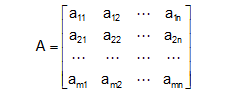or         A = [aij]m×n, 1 ≤ i ≤ m, 1 ≤ j ≤ n i, j ∈ N

Thus the ith row consists of the elements ai1, ai2, ai3, ..., ain, while the jth column consists of the elements a1j, a2j, a3j, ..., amj,

In general aij, is an element lying in the ith row and jth column. We can also read it as the (i, j)th element of A. The number of elements in an m × n matrix will be equal to mn.

Need Expert Assistance in IIT JAM Mathematics Preparation? Register Here for FREE & Get Counselling from Eduncle Experts!

## Determinant

To every square matrix A = [aij] of order n, we can associate a number (real or complex) called determinant of the matrix A, written as det A, where aij is the (i, j)th element of A.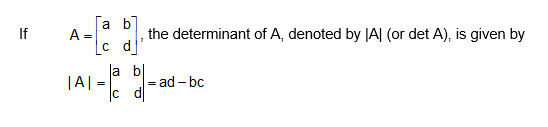NOTE:

(i)         Only square matrices have determinants.

(ii)        For a matrix A, |A| is called determinant of A and not modulus of A.

Determinant of a Matrix of Order One

Let A = [a] be the matrix of order 1, then determinant of A is defined to be equal to a.

Determinant of a Matrix of Order Two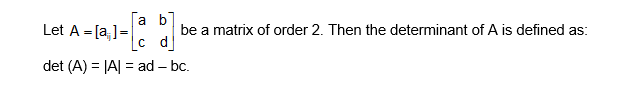Determinant of a Matrix of Order Three

The determinant of a matrix of order three can be determined by expressing it in terms of second order determinants which is known as expansion of determinant along a row (or a column). There are six way of expanding a determinant of order 3 corresponding to each of three rows (R1, R2 and R3) and three columns (C1, C2 and C3) and each way gives the same value.

Consider the determinant of a square matrix A = [aij]3×3, i.e.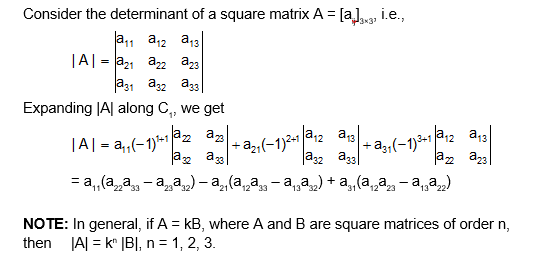Download the study notes for Determinant along with solved examples and practice questions here:## Eigen values and Eigen vectors

Definitions:

Let A = [aij]n×n be a square matrix of order n, I is an unit matrix of order n and λ an indeterminate, then the matrix.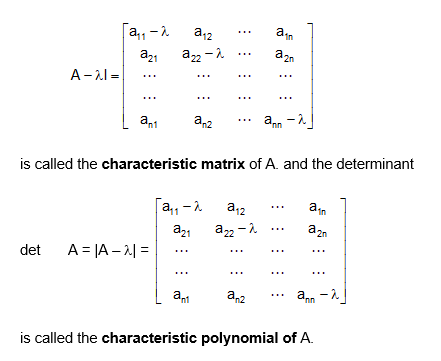Also the equation |A – λ| = 0, is called the characteristic equation of A. The roots of this equations are called the characteristic roots or characteristic value or Eigen roots or Eigen values of latent roots of the matrix.

The set of the Eigen values of the matrix A is called the spectrum of the matrix A. If λ is a characteristic root of a n × n matrix A, then the non-zero solutionof the equation A X = λ X i.e. (A – λ|) X = 0 is called the characteristic vector or Eigen vector of the matrix A corresponding to the characteristic root λ.

Chief Characteristics of Eigen Values:

The sum of the elements of the principal diagonal of a matrix is called the trace of the matrix.

(i)         Any matrix A and its transpose both have the same Eigen values.

(ii)        The trace of the matrix equals to the sum of the Eigen values of a matrix.

(iii)       The determinant of the matrix A equals to the product of the Eigen values of A.

(iv)       If λ1, λ2, ....., λn are the n-Eigen values of A, thenDo you want to Crack IIT JAM Mathematics 2020? With Over 1000+ Selections Eduncle is the First & Foremost Choice of Our Toppers. Join Eduncle.com for JAM Preparation & Create Your Own Success Story.

Some important Theorems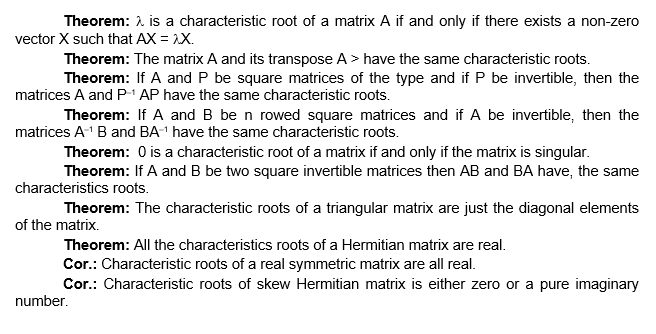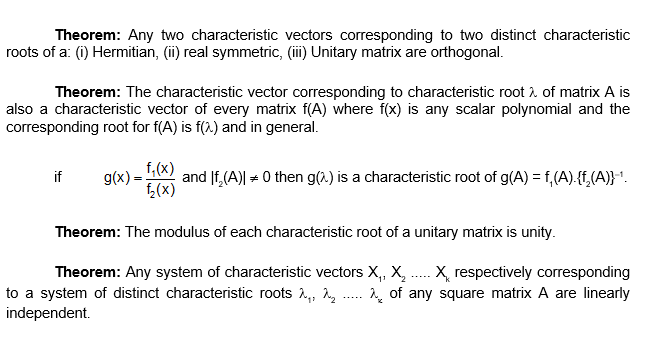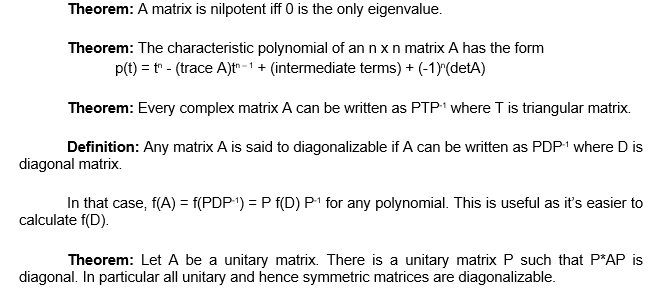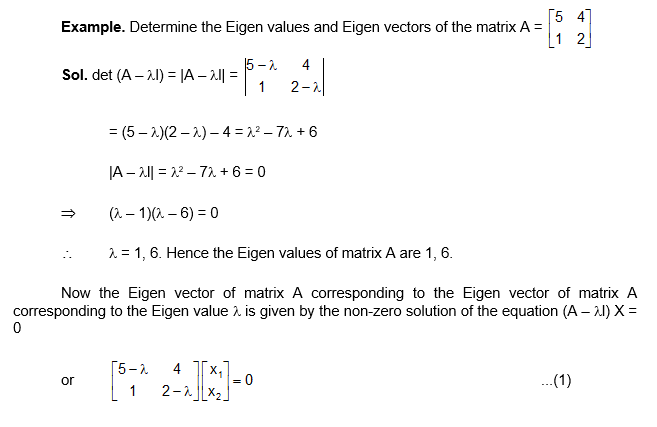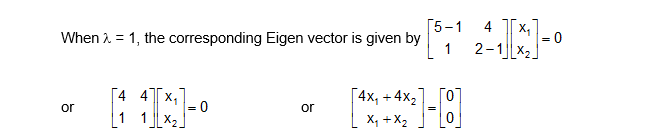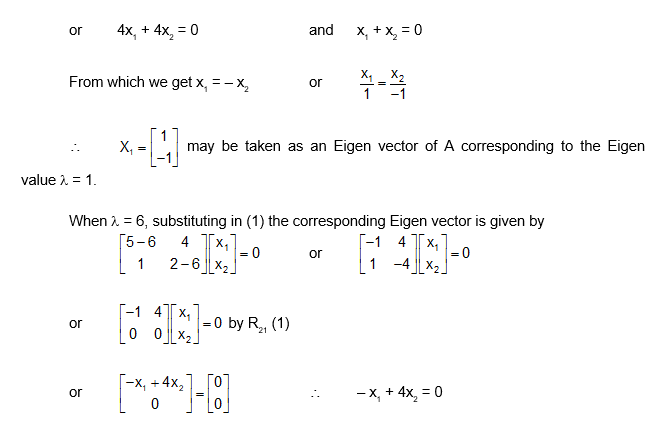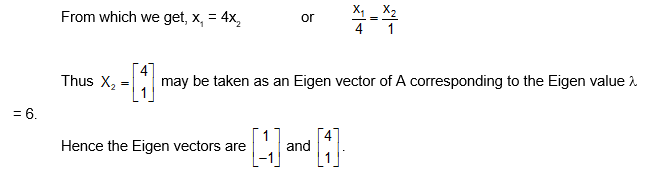### Cayley Hamilton Theorem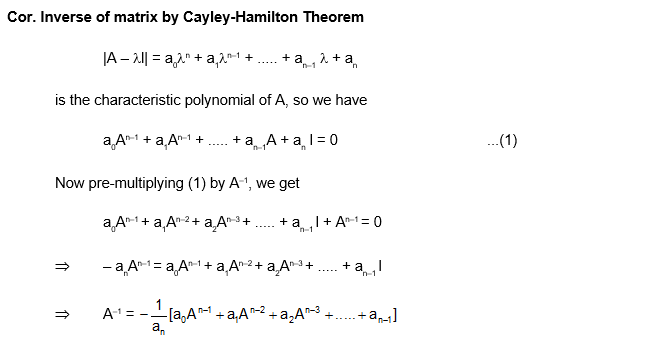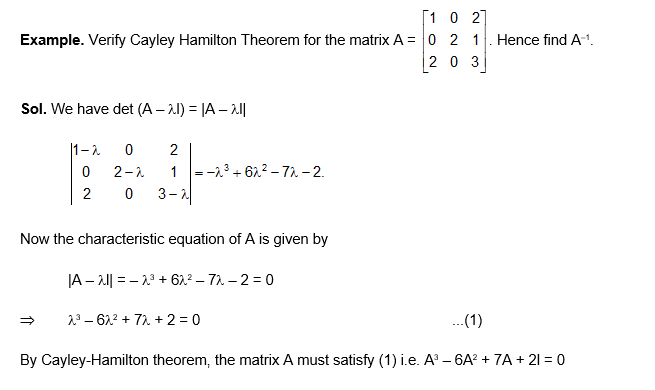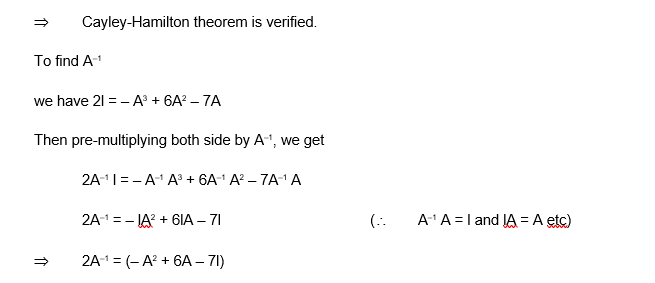## Practice Questions for Matrix and Determinants

Download the Practice Question PDF for Matrix and Determinants and get the solutions for all the below mentioned questions.### Basic Level Questions on Matrix and Determinant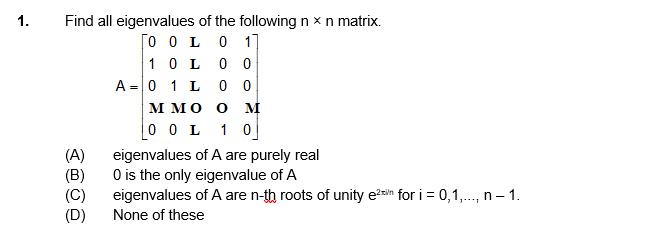Multiple Selection Question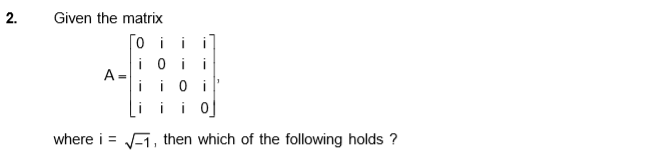Numerical Type Question### Challenging Questions on Matrix and Determinant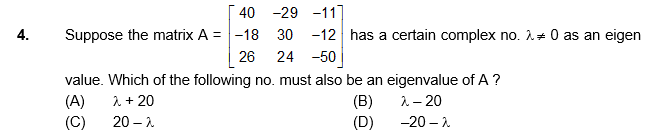Numerical Type Question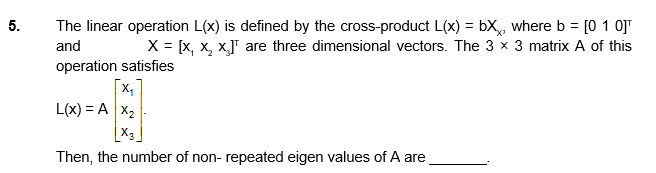Multiple Selection Question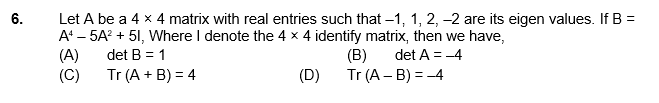### Previous Year Questions on Matrix and Determinant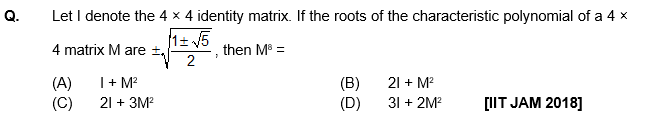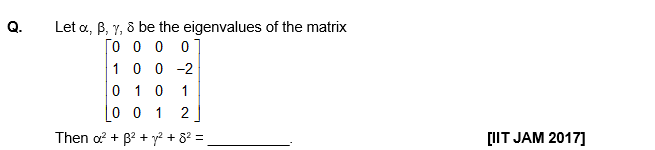View Solution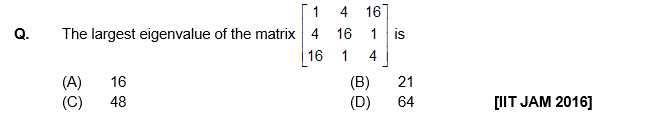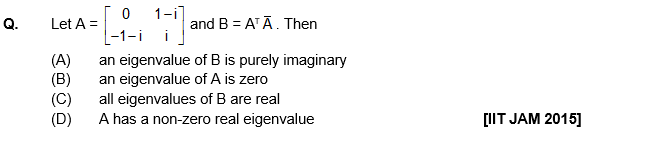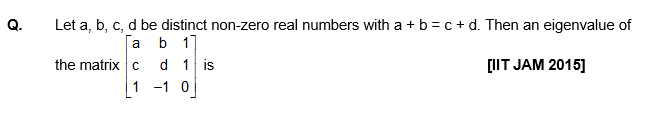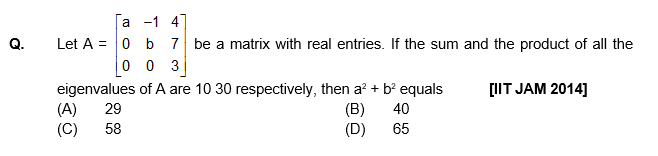Get reliable IIT JAM Mathematics Study Material with Previous Years Question Papers and Mock Test Papers. Download now!

### Related Topics:

Matrix and operations

Inverse of Matrix

Trace of a Matrix

Determinant

Minimal Polynomial

Type of Matrices

Algebraic and geometric multiplicity.

### FAQ:

What is the difference between matrix and determinants?

Key Difference: A matrix or matrices is a rectangular grid of numbers or symbols that is represented in a row and column format. A determinant is a component of a square matrix and it cannot be found in any other type of matrix.

Who invented matrix and determinants? Carl Gauss (1777-1855), the greatest German mathematician of the 19th century, first used the term 'determinant' in 1801. Matrices began in the 2nd century BC with the Chinese although traces could be seen back in the 4th century BC with the Babylonians.  It was only towards the end of the 17th century that much progress was made on the studies of matrices. Read more here – Matrix Mathematics

You Might Also Like